Home > A2C > Chapter 7 > Lesson 7.3.4 > Problem7-200

7-200.
1. Let A and B be the matrices defined below. Complete each of the following matrix arithmetic problems. Homework Help ✎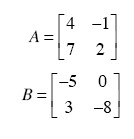1. A − B

2. 5A

3. AB

4. BA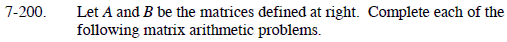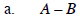$\begin{bmatrix} 9 & -1\\ 4 & 10 \end{bmatrix}$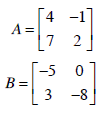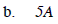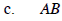$\begin{bmatrix} -23& \ 8\\ -29&-16 \end{bmatrix}$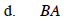Refer to parts (a), (b),and (c).